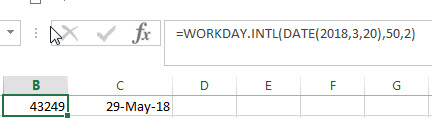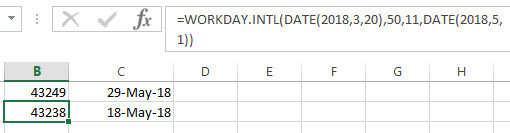# Excel WORKDAY.INTL Function

This post will guide you how to use Excel WORKDAY.INTL function with syntax and examples in Microsoft excel.

### Description

The Excel WORKDAY.INTL function returns the serial number of the date before or after a specified number of workdays. And this function allow you to exclude weekends and holidays as non-working days. You can use the WORKDAY.INTL function to calculate working days excluding weekdays and holidays.

The WORKDAY.INTL function is a build-in function in Microsoft Excel and it is categorized as a DATE and TIME Function.

The WORKDAY.INTL function is available in Excel 2016, Excel 2013, Excel 2010, Excel 2007, Excel 2003, Excel XP, Excel 2000, Excel 2011 for Mac.

### Syntax

The syntax of the WORKDAY.INTL function is as below:

`= WORKDAY.INTL (start_date, days, [weekend], [holidays])`

Where the WORKDAY.INTL function arguments is:

• Start_date –This is a required argument. The starting date from which you want to count the number of working days. The date should be typed as a valid Excel time a serial date.
• days – This is a required argument. The number of working days that you want to add. A positive value for days yields a future date; a negative value yields a past date.
• Weekend – This is an optional argument. Determine which days of the week that are weekend days and are not considered working days. Weekend is a weekend number and it can be one of the following numbers.
 weekend-number Weekend days 1 or omitted Saturday, Sunday 2 Sunday, Monday 3 Monday, Tuesday 4 Tuesday, Wednesday 5 Wednesday, Thursday 6 Thursday, Friday 7 Friday, Saturday 11 Sunday only 12 Monday only 13 Tuesday only 14 Wednesday only 15 Thursday only 16 Friday only 17 Saturday only
• holidays – This is an optional argument. The list of holidays that you want to exclude from the working days. It can be a range of cells that contain the holiday dates or it can be a list of serial numbers that represent the holiday dates.

Note:

• If any argument is not a valid Excel date, a #VALUE! Error is returned.
• If start date value plus days is an invalid date, the WORKDAY function returns #NUM! Error.
• A serial date is how the Microsoft Excel stores dates and it represents the number of days since 1900-01-01, so the January 1, 1900 date is serial number 1 by default.
• If days value is not a integer number, it will be truncated.
• If a weekend string is of invalid length or contains invalid characters, WORKDAY.INTL returns the #VALUE! Error value.

### Excel WORKDAY.INTL Function Examples

The below examples will show you how to use Excel WORKDAY.INTL Function to return the working days from the start date.

1# get the date 50 workdays from the starting date “3/20/2018”, counting Sunday and Monday as a weekend day, so the weekend argument should be 2, enter the following formula in Cell B1.

`=WORKDAY.INTL(DATE(2018,3,20),50,2)`2# get the date 50 workdays from the starting date “3/20/2018”, excluding holidays 5/1/2018, and counting only Sundays as weekend day, the weekend argument should be 11, type the following formula in Cell B2.

`=WORKDAY(DATE(2018,3,20),50,11,DATE(2018,5,1))`### Related Functions

• Excel WORKDAY function
The Excel WORKDAY function returns a serial number that represents a date that is the indicated number of working days before or after the starting date you specified.The syntax of the WORKDAY function is as below:=WORKDAY(start_date, days, [holidays])…
• Excel WEEKDAY function
The Excel WEEKDAY function returns a integer value representing the day fo the week for a given Excel date and the value is range from 1 to 7.The syntax of the WEEKDAY function is as below:=WEEKDAY (serial_number,[return_type])…
• Excel DAY function
The Excel DAY function returns a day of a date (from 1 to 31).The DAY function is a build-in function in Microsoft Excel and it is categorized as a DATE and TIME Function.The syntax of the DAY function is as below:= DAY (date_value)…
• Excel DATE function
The Excel DATE function returns the serial number for a date.The syntax of the DATE function is as below:= DATE (year, month, day)…
Related Posts

Calculating Future Date

This post will guide you how to calculate a future date based on a given data in excel. How do I add one or more days to a given date to calculate a future date with a formula in excel. ...

Excel DSTDEV Function

This post will guide you how to use Excel DSTDEV function with syntax and examples in Microsoft excel. Description The Excel DSTDEV returns the standard deviation of a population based on a sample of numbers in a column or database ...

Excel DMIN Function

This post will guide you how to use Excel DMIN function with syntax and examples in Microsoft excel. Description The Excel DMIN returns the minimum value from a database or a column of a list that matches the specified conditions. ...

Excel DPRODUCT Function

This post will guide you how to use Excel DPRODUCT function with syntax and examples in Microsoft excel. Description The Excel DPRODUCT returns the product of values from a set of records that match criteria that you specify. The DPRODUCT ...

Excel XIRR Function

This post will guide you how to use Excel XIRR function with syntax and examples in Microsoft excel. Description The Excel XIRR function returns the internal rate of return for a series of cash flows that is not necessarily periodic. ...

Excel VDB Function

This post will guide you how to use Excel VDB function with syntax and examples in Microsoft excel. Description The Excel VDB function calculates the depreciation of an asset for a specified period based on the double declining balance method. ...

Excel SYD Function

This post will guide you how to use Excel SYD function with syntax and examples in Microsoft excel. Description The Excel SYD function calculates the sum-of-years’digits depreciation of an asset for a specified period. And it will return a numeric ...

Excel SLN Function

This post will guide you how to use Excel SLN function with syntax and examples in Microsoft excel. Description The Excel SLN function calculates the depreciation of an asset for one period based on the straight line depreciation. And it ...

Excel PV Function

This post will guide you how to use Excel PV function with syntax and examples in Microsoft excel. Description The Excel PV function returns the present value of a loan or investment based on constant payments and a constant interest ...

Excel RATE Function

This post will guide you how to use Excel RATE function with syntax and examples in Microsoft excel. Description The Excel RATE function returns the interest rate per payment period of an annuity. The RATE function is a build-in function ...

Sidebar